• dd_engi背包九讲的个人整理，包含代码例题及个人理解
DD engi 背包九讲的个人整理
写在前面
这篇文章涵盖了dd_engi背包九讲的所有内容，基本框架相同，但加上了许多个人的理解、详细解释、代码实现与例题，去除了我认为没有多大用的伪代码替换成了好理解的代码，字母都加上了$LaTeX$，采用相同的变量，相信能让读者理解透彻，不用再翻那么多博客反复学习。
文章最后有我自己整理的各种背包的综合模板，可供参考。如有错误劳烦指出。
背包九讲最新版pdf（beta1.2 20120508）已由作者崔添翼（dd_engi）上传至github，完全公开，不需要在其他网站花费积分甚至付费下载，github下载链接 网盘 633c01背包问题
题目
有$N$件物品和一个容量为$V$的背包。第$i$件物品的费用是$w[i]$，价值是$v[i]$，求将哪些物品装入背包可使价值总和最大。
基本思路
这是最基础的背包问题，特点是：每种物品仅有一件，可以选择放或不放。
用子问题定义状态：即$f[i][j]$表示前$i$件物品恰放入一个容量为$j$的背包可以获得的最大价值。则其状态转移方程便是：

$f[i][j]=max({f[i-1][j],f[i-1][j-w[i]]+v[i]})$

这个方程非常重要，基本上所有跟背包相关的问题的方程都是由它衍生出来的。所以有必要将它详细解释一下：“将前$i$件物品放入容量为$j$的背包中”这个子问题，若只考虑第$i$件物品的策略（放或不放），那么就可以转化为一个只牵扯前$i-1$件物品的问题。如果不放第$i$件物品，那么问题就转化为“前$i-1$件物品放入容量为$j$的背包中”，价值为$f[i-1][j]$；如果放第$i$件物品，那么问题就转化为“前$i-1$件物品放入剩下的容量为$j-w[i]$的背包中”，此时能获得的最大价值就是$f[i-1][j-w[i]]$再加上通过放入第$i$件物品获得的价值$v[i]$。
优化空间复杂度
以上方法的时间和空间复杂度均为$O(VN)$，其中时间复杂度已经不能再优化了，但空间复杂度却可以优化到$O(N)$。
先考虑上面讲的基本思路如何实现，肯定是有一个主循环$i=1...N$，每次算出来二维数组$f[i][0...V]$的所有值。那么，如果只用一个数组$f[0...V]$，能不能保证第$i$次循环结束后$f[j]$中表示的就是我们定义的状态$f[i][j]$呢？$f[i][j]$是由$f[i-1][j]$和$f[i-1][j-w[i]]$两个子问题递推而来，能否保证在推$f[i][j]$时（也即在第$i$次主循环中推$f[j]$时）能够得到$f[i-1][j]$和$f[i-1][j-w[i]]$的值呢？事实上，这要求在每次主循环中我们以$j=V...0$的顺序推$f[j]$，这样才能保证推$f[j]$时$f[j-w[i]]$保存的是状态$f[i-1][j-w[i]]$的值。至于为什么下面有详细解释。代码如下：
​for (int i = 1; i <= n; i++)
for (int j = V; j >= 0; j--)
f[j] = max(f[j], f[j - w[i]] + v[i]);

其中的$f[j]=max({f[j],f[j-w[i]]})$一句恰就相当于我们的转移方程$f[i][j]=max({f[i-1][j],f[i-1][j-w[i]]})$，因为现在的$f[j-w[i]]$就相当于原来的$f[i-1][j-w[i]]$。如果将$V$的循环顺序从上面的逆序改成顺序的话，那么则成了$f[i][j]$由$f[i][j-w[i]]$推知，与本题意不符，但它却是另一个重要的背包问题（完全背包）最简捷的解决方案，故学习只用一维数组解01背包问题是十分必要的。
事实上，使用一维数组解01背包的程序在后面会被多次用到，以后的代码中就直接调用不再加以说明。
下面再给出一段代码，注意和上面的代码有什么不同
​for (int i = 1; i <= n; i++)
for (int j = V; j >= w[i]; j--)
f[j] = max(f[j], f[j - w[i]] + v[i]);

注意这个过程里的处理与前面给出的代码有所不同。前面的示例程序写成$j=V...0$是为了在程序中体现每个状态都按照方程求解了，避免不必要的思维复杂度。而这里既然已经抽象成看作黑箱的过程了，就可以加入优化。费用为$w[i]$的物品不会影响状态$f[0...j-1]$，这是显然的。
初始化的细节问题
我们看到的求最优解的背包问题题目中，事实上有两种不太相同的问法。有的题目要求"恰好装满背包"时的最优解，有的题目则并没有要求必须把背包装满。这两种问法的区别是在初始化的时候有所不同。
如果是第一种问法，要求恰好装满背包，那么在初始化时除了$f$为$0$其它$f[1...V]$均设为$-∞$，这样就可以保证最终得到的$f[N]$是一种恰好装满背包的最优解。
如果并没有要求必须把背包装满，而是只希望价格尽量大，初始化时应该将$f[0...V]$全部设为$0$。
为什么呢？可以这样理解：初始化的$f$数组事实上就是在没有任何物品可以放入背包时的合法状态。如果要求背包恰好装满，那么此时只有容量为$0$的背包可能被价值为$0$的$nothing$“恰好装满”，其它容量的背包均没有合法的解，属于未定义的状态，它们的值就都应该是$-∞$了。如果背包并非必须被装满，那么任何容量的背包都有一个合法解“什么都不装”，这个解的价值为$0$，所以初始时状态的值也就全部为$0$了。这个小技巧完全可以推广到其它类型的背包问题，后面也就不再对进行状态转移之前的初始化进行讲解。
一个常数优化
前面的代码中有$for(j=V...w[i])$，还可以将这个循环的下限进行改进。
由于只需要最后$f[j]$的值，倒推前一个物品，其实只要知道$f[j-w[n]]$即可。以此类推，对以第$j$个背包，其实只需要知道到$f[j-sum({w[j...n]})]$即可，即代码可以改成
for (int i = 1; i <= n; i++) {
int bound = max(V - sum{w[i + 1]...w[n]}, w[i]);
for (int j = V; j >= bound, j--)
f[j] = max(f[j], f[j - w[i]] + v[i]);
}

对于求$sum$可以用前缀和，这对于$V$比较大时是有用的。
具体代码：
for (int i = 1; i <= n; i++) cin >> w[i] >> v[i], s[i] = s[i - 1] + w[i];
for (int i = 1; i <= n; i++) {
int bound = max(w[i], V - (s[n] - s[i]));
for (int j = V; j >= bound; j--)
f[j] = max(f[j], f[j - w[i]] + v[i]);
}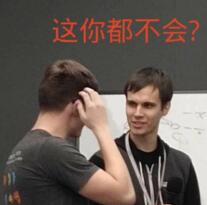小结
01背包问题是最基本的背包问题，它包含了背包问题中设计状态、方程的最基本思想，另外，别的类型的背包问题往往也可以转换成01背包问题求解。故一定要仔细体会上面基本思路的得出方法，状态转移方程的意义，以及最后怎样优化的空间复杂度。
例题：
Luogu 2925 干草出售
Luogu 1616 疯狂的采药
HDU 3466 Proud Merchants
完全背包问题
题目
有$N$种物品和一个容量为$V$的背包，每种物品都有无限件可用。第$i$种物品的费用是$w[i]$，价值是$v[i]$。求解将哪些物品装入背包可使这些物品的费用总和不超过背包容量，且价值总和最大。
基本思路
这个问题非常类似于01背包问题，所不同的是每种物品有无限件。也就是从每种物品的角度考虑，与它相关的策略已并非取或不取两种，而是有取$0$件、取$1$件、取$2$件……等很多种。如果仍然按照解01背包时的思路，令$f[i][j]$表示前$i$种物品恰放入一个容量为$V$的背包的最大权值。仍然可以按照每种物品不同的策略写出状态转移方程，像这样：

$f[i][j]=max({f[i-1][j-k*w[i]]+k*v[i])|0<=k*w[i]<=j}$

这跟01背包问题一样有$O(VN)$个状态需要求解，但求解每个状态的时间已经不是常数了，求解状态$f[i][j]$的时间是$O(V/w[i])$，总的复杂度可以认为是$O(N*Σ(V/w[i]))$，是比较大的。将01背包问题的基本思路加以改进，得到了这样一个清晰的方法。这说明01背包问题的方程的确是很重要，可以推及其它类型的背包问题。但我们还是试图改进这个复杂度。
一个简单有效的优化
完全背包问题有一个很简单有效的优化，是这样的：若两件物品$i$、$j$满足$w[i]<=w[j]$且$v[i]>=v[j]$，则将物品$j$去掉，不用考虑。这个优化的正确性显然：任何情况下都可将价值小费用高得$j$换成物美价廉的$i$，得到至少不会更差的方案。对于随机生成的数据，这个方法往往会大大减少物品的件数，从而加快速度。然而这个并不能改善最坏情况的复杂度，因为有可能特别设计的数据可以一件物品也去不掉。
这个优化可以简单的$O(N^{2})$地实现，一般都可以承受。另外，针对背包问题而言，比较不错的一种方法是：首先将费用大于$V$的物品去掉，然后使用类似计数排序的做法，计算出费用相同的物品中价值最高的是哪个，可以$O(V+N)$地完成这个优化。转化为01背包问题求解
既然01背包问题是最基本的背包问题，那么我们可以考虑把完全背包问题转化为01背包问题来解。最简单的想法是，考虑到第$i$种物品最多选$V/w[i]$件，于是可以把第$i$种物品转化为$V/w[i]$件费用及价值均不变的物品，然后求解这个01背包问题。这样完全没有改进基本思路的时间复杂度，但这毕竟给了我们将完全背包问题转化为01背包问题的思路：将一种物品拆成多件物品。
更高效的转化方法是：把第$i$种物品拆成费用为$w[i]*2^{k}$、价值为$v[i]*2^{k}$的若干件物品，其中$k$满足$w[i]*2^{k}<=V$。这是二进制的思想，因为不管最优策略选几件第$i$种物品，总可以表示成若干个$2^{k}$件物品的和。这样把每种物品拆成$O(log (V/w[i]))$件物品，是一个很大的改进。但我们有更优的$O(VN)$的算法。
$O(VN)$的算法
这个算法使用一维数组，先看代码：
for (int i = 1; i <= n; i++)
for (int j = w[i]; j <= V; j++)
f[j] = max(f[j], f[j - w[i]] + v[i]);

细心的读者会发现，这个代码与01背包的代码只有$j$的循环次序不同而已。为什么这样一改就可行呢？首先想想为什么01背包中要按照$j=V...0$的逆序来循环。这是因为要保证第$i$次循环中的状态$f[i][j]$是由状态$f[i-1][j-w[i]]$递推而来。换句话说，这正是为了保证每件物品只选一次，保证在考虑“选入第$i$件物品”这件策略时，依据的是一个绝无已经选入第$i$件物品的子结果$f[i-1][j-w[i]]$。而现在完全背包的特点恰是每种物品可选无限件，所以在考虑“加选一件第$i$种物品”这种策略时，却正需要一个可能已选入第$i$种物品的子结果$f[i][j-w[i]]$，所以就可以并且必须采用$j=0...V$的顺序循环。这就是这个简单的程序为何成立的道理。值得一提的是，上面的伪代码中两层$for$循环的次序可以颠倒。这个结论有可能会带来算法时间常数上的优化。
这个算法也可以以另外的思路得出。例如，将基本思路中求解$f[i][j-w[i]]$的状态转移方程显式地写出来，代入原方程中，会发现该方程可以等价地变形成这种形式：

$f[i][j]=max({f[i-1][j],f[i][j-w[i]]+v[i]})$

将这个方程用一维数组实现，便得到了上面的代码。
小结
完全背包问题也是一个相当基础的背包问题，它有两个状态转移方程，分别在“基本思路”以及“$O(VN)$的算法“的小节中给出。希望你能够对这两个状态转移方程都仔细地体会，不仅记住，也要弄明白它们是怎么得出来的，最好能够自己想一种得到这些方程的方法。事实上，对每一道动态规划题目都思考其方程的意义以及如何得来，是加深对动态规划的理解、提高动态规划功力的好方法。
例题：
HDU 1114 Piggy-Bank
Luogu 1853 投资的最大效益
顺序枚举与倒序枚举详细解释
首先说完全背包的正序枚举
假设你现在有一个体积为$V$的背包，有一个体积为$3$，价值为$4$的物品，如果正序枚举的话，等我们填充到$3$这个位置，就会得到价值为4的物品，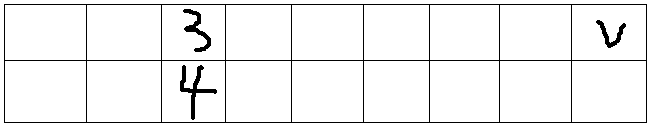等我们在填充到$6$这个位置时，发现还可以造成更大的价值，也就是把这个物品再用一遍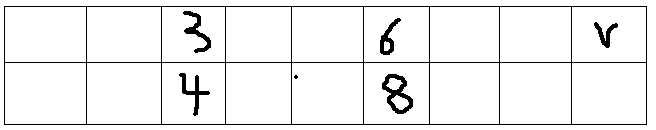这就是完全背包为什么体积正序枚举的原因
下面说01背包的倒序枚举
题目一样，此时倒序填充背包，为了方便从$6$开始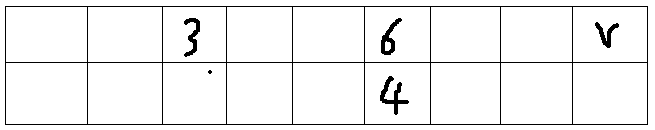此时，在体积为6的背包中有了价值为4的物品，但体积为3的背包中物品的价值仍然为0，也就是说填充体积为6的背包只得到了填充体积为3的背包的价值，等再枚举到体积为3的背包时，我们才填充了体积为3的背包，接下来枚举物品是就不会再重复使用这个物品了，所以这个物品只被放了一次，最后的状态是这样的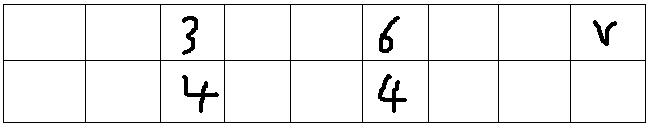也就是为什么01背包要倒序枚举的原因。
还没看明白的话，还有一篇更详细的——点这里
多重背包问题
题目
有$N$种物品和一个容量为$V$的背包。第$i$种物品最多有$p[i]$件可用，每件费用是$w[i]$，价值是$v[i]$。求解将哪些物品装入背包可使这些物品的费用总和不超过背包容量，且价值总和最大。
基本算法
这题目和完全背包问题很类似。基本的方程只需将完全背包问题的方程略微一改即可，因为对于第$i$种物品有$p[i]+1$种策略：取$0$件，取$1$件……取$p[i]$件。令$f[i][j]$表示前$i$种物品恰放入一个容量为$j$的背包的最大权值，则有状态转移方程：

$f[i][j]=max({f[i-1][j-k*w[i]]+k*v[i])|0<=k<=p[i]}$

复杂度是$O(V*Σp[i])$。
转化为01背包问题
另一种好想好写的基本方法是转化为01背包求解：把第$i$种物品换成$p[i]$件01背包中的物品，则得到了物品数为$Σp[i]$的01背包问题，直接求解，复杂度仍然是$O(V*Σp[i])$。但是我们期望将它转化为01背包问题之后能够像完全背包一样降低复杂度。仍然考虑二进制的思想，我们考虑把第$i$种物品换成若干件物品，使得原问题中第$i$种物品可取的每种策略——取$0...p[i]$件——均能等价于取若干件代换以后的物品。另外，取超过$p[i]$件的策略必不能出现。
方法是：将第$i$种物品分成若干件物品，其中每件物品有一个系数，这件物品的费用和价值均是原来的费用和价值乘以这个系数。使这些系数分别为$1,2,4,...,2^{k-1},p[i]-2^{k}+1$，且$k$是满足$p[i]-2^{k}+1>0$的最大整数。例如，如果$p[i]$为$13$，就将这种物品分成系数分别为$1,2,4,6$的四件物品。分成的这几件物品的系数和为$p[i]$，表明不可能取多于$p[i]$件的第$i$种物品。另外这种方法也能保证对于$0...p[i]$间的每一个整数，均可以用若干个系数的和表示，这个证明可以分$0...2^{k}-1$和$2^{k}...p[i]$两段来分别讨论得出，并不难，希望你自己思考尝试一下。这样就将第$i$种物品分成了$O(log(p[i]))$种物品，将原问题转化为了复杂度为$O(V*Σlog(p[i]))$的01背包问题，是很大的改进。二进制拆分代码如下：
for (int i = 1; i <= n; i++) {
int num = min(p[i], V / w[i]);
for (int k = 1; num > 0; k <<= 1) {
if (k > num) k = num;
num -= k;
for (int j = V; j >= w[i] * k; j--)
f[j] = max(f[j], f[j - w[i] * k] + v[i] * k);
}
}

O(VN)的算法
实际情况下二进制拆分已经够用，不会有人把时间卡到只能用单调队列优化，下面的优化看不懂的同学不要强求！
多重背包问题同样有$O(VN)$的算法。这个算法基于基本算法的状态转移方程，但应用单调队列的方法使每个状态的值可以以均摊$O(1)$的时间求解。代码如下（需要外套$1...n$循环）：
//p：某类物品数量，w：某类物品花费，v：某类物品价值，V:商品总价值
void MultiPack(int p, int w, int v) {
for (int j = 0; j < w; j++) { //j为w的所有组
int head = 1, tail = 0;
for (int k = j, i = 0; k <= V / 2; k += w, i++) {
int r = f[k] - i * v;
while (head <= tail and r >= q[tail].v) tail--;
q[++tail] = node(i, r);
while (q[head].id < i - p) head++; //需要的物品数目
f[k] = q[head].v + i * v;
}
}
}

这里原作者并没有作过多解释，代码也没有给，应要求在这里讲一下，是个人的之前理解
此前应先确保搞明白了单调队列，就是在区间移动时动态维护区间的最值
观察它的转移方程：$f[i][j]=max({f[i-1][j],f[i-1][j-k*w[i]]+k*v[i])}$
单调队列优化的主要思想就是分组更新，因为$w[i]$是成倍增加的
$f[i-1][j]$只会更新$f[i-1][j+k*w[i]]$（这里是从前往后看的，所以是+）
对于当前为$w$的体积，我们可以按照余数将它分为$w$组，也就是$0...w-1$
同一个剩余系的数在一组
比如在模$3$意义下，$1,4,7,10$是一组，$2,5,8,11$是一组，$3,6,9,12$是一组
每组的转移是互不影响的，也就是单独转移
举个例子
$f[i][5w]=max({f[i-1][4w]+w,f[i-1][3w]+2v,f[i-1][2w]+3v,f[i-1][w]+4v,f[i-1]+5v})$
$f[i][4w]=max({f[i-1][3w]+w,f[i-1][2w]+2v,f[i-1][w]+3v,f[i-1]+4v})$
让所有的$f[i][j]$都减去$j/w*v$，式子就变成
$f[i][5w]=max({f[i-1][4w]-4v,f[i-1][3w]-3v,f[i-1][2w]-2v,f[i-1][w]-v,f[i-1]})$
$f[i][4w]=max({f[i-1][3w]-3v,f[i-1][2w]-2v,f[i-1][w]-v,f[i-1]})$
即$f[i][j]=max({f[i-1][j\mod w+k*w]-k*v}+j*v)$
当$j\mod w$一定后，就可以用单调队列来优化了小结
这里我们看到了将一个算法的复杂度由$O(V*Σp[i])$改进到$O(V*Σlog(p[i]))$的过程，还知道了存在应用超出$NOIP$范围的知识的$O(VN)$算法。希望你特别注意"拆分物品"的思想和方法，自己证明一下它的正确性，并将完整的程序代码写出来。
例题：
HDU 1059 Dividing
Luogu P1776 宝物筛选
混合背包问题
问题
如果将前面三个背包混合起来，也就是说，有的物品只可以取一次（01背包），有的物品可以取无限次（完全背包），有的物品可以取的次数有一个上限（多重背包），应该怎么求解呢？
01背包与完全背包的混合
考虑到在01背包和完全背包中给出的伪代码只有一处不同，故如果只有两类物品：一类物品只能取一次，另一类物品可以取无限次，那么只需在对每个物品应用转移方程时，根据物品的类别选用顺序或逆序的循环即可，复杂度是$O(VN)$。
再加上多重背包
如果再加上有的物品最多可以取有限次，那么原则上也可以给出$O(VN)$的解法：遇到多重背包类型的物品用单调队列解即可。但如果不考虑超过$NOIP$范围的算法的话，用多重背包中将每个这类物品分成$O(log(p[i]))$个01背包的物品的方法也已经很优了。当然，更清晰的写法是调用我们前面给出的三个相关过程。代码：
p[i]:每个物品的件数，0代表无穷个
for (int i = 1; i <= n; i++)
if (p[i] == 0)
for (int j = w[i]; j <= V; j++)
f[j] = max(f[j], f[j - w[i]] + v[i]);
else
for (int k = 1; k <= p[i]; k++)
for (int j = V; j >= w[i]; j--)
f[j] = max(f[j], f[j - w[i]] + v[i]);

在最初写出这三个过程的时候，可能完全没有想到它们会在这里混合应用。这就体现了编程中抽象的威力。如果你一直就是以这种"抽象出过程"的方式写每一类背包问题的，也非常清楚它们的实现中细微的不同，那么在遇到混合三种背包问题的题目时，一定能很快想到上面简洁的解法。
小结
有人说，困难的题目都是由简单的题目叠加而来的。这句话是否公理暂且存之不论，但它在本讲中已经得到了充分的体现。本来01背包、完全背包、多重背包都不是什么难题，但将它们简单地组合起来以后就得到了这样一道一定能吓倒不少人的题目。但只要基础扎实，领会三种基本背包问题的思想，就可以做到把困难的题目拆分成简单的题目来解决。
例题：
Luogu P1833 樱花
HDU 3535 AreYouBusy
二维费用背包问题
问题
二维费用的背包问题是指：对于每件物品，具有两种不同的费用；选择这件物品必须同时付出这两种代价；对于每种代价都有一个可付出的最大值（背包容量）。问怎样选择物品可以得到最大的价值。设这两种代价分别为代价$1$和代价$2$，第$i$件物品所需的两种代价分别为$w[i]$和$g[i]$。两种代价可付出的最大值（两种背包容量）分别为$V$和$T$。物品的价值为$v[i]$。
算法
费用加了一维，只需状态也加一维即可。设$f[i][j][k]$表示前$i$件物品付出两种代价分别为$j$和$k$时可获得的最大价值。状态转移方程就是：$f[i][j][k]=max({f[i-1][j][k],f[i-1][j-w[i]][k-g[i]]+v[i]})$
如前述方法，可以只使用二维的数组：当每件物品只可以取一次时变量$j$和$k$采用逆序的循环，当物品有如完全背包问题时采用顺序的循环。当物品有如多重背包问题时拆分物品。代码：
for (int i = 1; i <= n; i++)
for (int j = V; j >= w[i]; j--)
for (int k = T; k >= g[i]; k--)
f[j][k] = max(f[j][k], f[j - w[i]][k - g[i]] + v[i]);

物品总个数的限制
有时，“二维费用”的条件是以这样一种隐含的方式给出的：最多只能取$M$件物品。这事实上相当于每件物品多了一种“件数”的费用，每个物品的件数费用均为$1$，可以付出的最大件数费用为$M$。换句话说，设$f[i][j]$表示付出费用$i$、最多选$j$件时可得到的最大价值，则根据物品的类型（01、完全、多重）用不同的方法循环更新，最后在$f[0...V][0...M]$范围内寻找答案。
复数域上的背包问题
另一种看待二维背包问题的思路是：将它看待成复数域上的背包问题。也就是说，背包的容量以及每件物品的费用都是一个复数。而常见的一维背包问题则是实数域上的背包问题。（注意：上面的话其实不严谨，因为事实上我们处理的都只是整数而已。）所以说，一维背包的种种思想方法，往往可以应用于二位背包问题的求解中，因为只是数域扩大了而已。
例题：
Luogu 1507 NASA的食物计划
HDU 2159 FATE
分组背包问题
问题
有$N$件物品和一个容量为$V$的背包。第i件物品的费用是$w[i]$，价值是$v[i]$。这些物品被划分为若干组，每组中的物品互相冲突，最多选一件。求解将哪些物品装入背包可使这些物品的费用总和不超过背包容量，且价值总和最大。
算法
这个问题变成了每组物品有若干种策略：是选择本组的某一件，还是一件都不选。也就是说设$f[k][j]$表示前$k$组物品花费费用$j$能取得的最大权值，则有：

$f[k][j]=max({f[k-1][j],f[k-1][j-c[i]]+w[i]|物品i属于组k})$

伪代码如下：
for (所有的组k)
for (int j = V; j >= 0; j--)
for (所有属于组k的i)
f[j] = max{f[j], f[j - w[i]] + v[i]}

注意这里的三层循环的顺序，$for (j...0)$这一层循环必须在$for$(所有的$i$属于组$k$)之外。这样才能保证每一组内的物品最多只有一个会被添加到背包中。
另外，显然可以对每组内的物品应用完全背包中“一个简单有效的优化”。
小结
分组的背包问题将彼此互斥的若干物品称为一个组，这建立了一个很好的模型。不少背包问题的变形都可以转化为分组的背包问题（例如有依赖的背包），由分组的背包问题进一步可定义“泛化物品”的概念，十分有利于解题。
例题：
Luogu 1757 通天之分组背包
HDU 1712 ACboy needs your help
有依赖背包问题
简化的问题
这种背包问题的物品间存在某种“依赖”的关系。也就是说，$i$依赖于$j$，表示若选物品$i$，则必须选物品$j$。为了简化起见，我们先设没有某个物品既依赖于别的物品，又被别的物品所依赖；另外，没有某件物品同时依赖多件物品。
算法
这个问题由$NOIP2006$金明的预算方案一题扩展而来。遵从该题的提法，将不依赖于别的物品的物品称为“主件”，依赖于某主件的物品称为“附件”。由这个问题的简化条件可知所有的物品由若干主件和依赖于每个主件的一个附件集合组成。
按照背包问题的一般思路，仅考虑一个主件和它的附件集合。可是，可用的策略非常多，包括：一个也不选，仅选择主件，选择主件后再选择一个附件，选择主件后再选择两个附件…无法用状态转移方程来表示如此多的策略。（事实上，设有$n$个附件，则策略有$2^{n}+1$个，为指数级。）
考虑到所有这些策略都是互斥的（也就是说，你只能选择一种策略），所以一个主件和它的附件集合实际上对应于分组背包中的一个物品组，每个选择了主件又选择了若干个附件的策略对应于这个物品组中的一个物品，其费用和价值都是这个策略中的物品的值的和。但仅仅是这一步转化并不能给出一个好的算法，因为物品组中的物品还是像原问题的策略一样多。
再考虑分组背包中的一句话： 可以对每组中的物品应用完全背包中“一个简单有效的优化”。 这提示我们，对于一个物品组中的物品，所有费用相同的物品只留一个价值最大的，不影响结果。
所以，我们可以对主件i的“附件集合”先进行一次01背包，得到费用依次为$0...V-c[i]$所有这些值时相应的最大价值$f'[0...V-c[i]]$。那么这个主件及它的附件集合相当于$V-c[i]+1$个物品的物品组，其中费用为$c[i]+k$的物品的价值为$f'[k]+w[i]$。也就是说原来指数级的策略中有很多策略都是冗余的，通过一次01背包后，将主件$i$转化为$V-c[i]+1$个物品的物品组，就可以直接应用分组背包的算法解决问题了。
较一般的问题
更一般的问题是：依赖关系以图论中“森林”的形式给出（森林即多叉树的集合），也就是说，主件的附件仍然可以具有自己的附件集合，限制只是每个物品最多只依赖于一个物品（只有一个主件）且不出现循环依赖。
解决这个问题仍然可以用将每个主件及其附件集合转化为物品组的方式。唯一不同的是，由于附件可能还有附件，就不能将每个附件都看作一个一般的01背包中的物品了。若这个附件也有附件集合，则它必定要被先转化为物品组，然后用分组的背包问题解出主件及其附件集合所对应的附件组中各个费用的附件所对应的价值。
事实上，这是一种树形$DP$，其特点是每个父节点都需要对它的各个儿子的属性进行一次$DP$以求得自己的相关属性。这已经触及到了“泛化物品”的思想。看完泛化物品后，你会发现这个“依赖关系树”每一个子树都等价于一件泛化物品，求某节点为根的子树对应的泛化物品相当于求其所有儿子的对应的泛化物品之和。
小结
拿金明的预算方案来说，通过引入“物品组”和“依赖”的概念可以加深对这题的理解，还可以解决它的推广问题。用物品组的思想考虑那题中极其特殊的依赖关系：物品不能既作主件又作附件，每个主件最多有两个附件，可以发现一个主件和它的两个附件等价于一个由四个物品组成的物品组，这便揭示了问题的某种本质。
例题：
HDU 3449 Consumer
Luogu 1064 金明的预算方案
泛化物品
定义
考虑这样一种物品，它并没有固定的费用和价值，而是它的价值随着你分配给它的费用而变化。这就是泛化物品的概念。
更严格的定义之。在背包容量为$V$的背包问题中，泛化物品是一个定义域为$0...V$中的整数的函数$h$，当分配给它的费用为v时，能得到的价值就是$h(v)$。
这个定义有一点点抽象，另一种理解是一个泛化物品就是一个数组$h[0...V]$，给它费用$v$，可得到价值$h[V]$。
一个费用为$c$价值为$w$的物品，如果它是01背包中的物品，那么把它看成泛化物品，它就是除了$h(c)=w$其它函数值都为$0$的一个函数。如果它是完全背包中的物品，那么它可以看成这样一个函数，仅当$v$被$c$整除时有$h(v)=v/c*w$，其它函数值均为$0$。如果它是多重背包中重复次数最多为$n$的物品，那么它对应的泛化物品的函数有$h(v)=v/c*w$仅当$v$被$c$整除且$v/c<=n$，其它情况函数值均为$0$。一个物品组可以看作一个泛化物品$h$。对于一个$0...V$中的$v$，若物品组中不存在费用为$v$的的物品，则$h(v)=0$，否则$h(v)$为所有费用为$v$的物品的最大价值。有依赖的背包问题中每个主件及其附件集合等价于一个物品组，自然也可看作一个泛化物品。
泛化物品的和
如果面对两个泛化物品$h$和$l$，要用给定的费用从这两个泛化物品中得到最大的价值，怎么求呢？事实上，对于一个给定的费用$v$，只需枚举将这个费用如何分配给两个泛化物品就可以了。同样的，对于$0...V$的每一个整数$v$，可以求得费用$v$分配到$h$和$l$中的最大价值$f(v)$。也即

$f(v)=max({h(k)+l(v-k)|0<=k<=v})$

可以看到，$f$也是一个由泛化物品$h$和$l$决定的定义域为$0...V$的函数，也就是说，$f$是一个由泛化物品$h$和$l$决定的泛化物品。
由此可以定义泛化物品的和：$h、l$都是泛化物品，若泛化物品$f$满足以上关系式，则称$f$是$h$与$l$的和。这个运算的时间复杂度取决于背包的容量，是$O(V^{2})$。
泛化物品的定义表明：在一个背包问题中，若将两个泛化物品代以它们的和，不影响问题的答案。事实上，对于其中的物品都是泛化物品的背包问题，求它的答案的过程也就是求所有这些泛化物品之和的过程。设此和为$s$，则答案就是$s[0...V]$中的最大值。
背包问题的泛化物品
一个背包问题中，可能会给出很多条件，包括每种物品的费用、价值等属性，物品之间的分组、依赖等关系等。但肯定能将问题对应于某个泛化物品。也就是说，给定了所有条件以后，就可以对每个非负整数$v$求得：若背包容量为$v$，将物品装入背包可得到的最大价值是多少，这可以认为是定义在非负整数集上的一件泛化物品。这个泛化物品——或者说问题所对应的一个定义域为非负整数的函数——包含了关于问题本身的高度浓缩的信息。一般而言，求得这个泛化物品的一个子域（例如$0...V$）的值之后，就可以根据这个函数的取值得到背包问题的最终答案。
综上所述，一般而言，求解背包问题，即求解这个问题所对应的一个函数，即该问题的泛化物品。而求解某个泛化物品的一种方法就是将它表示为若干泛化物品的和然后求之。
背包问题问法的变化
以上涉及的各种背包问题都是要求在背包容量（费用）的限制下求可以取到的最大价值，但背包问题还有很多种灵活的问法，在这里值得提一下。但是我认为，只要深入理解了求背包问题最大价值的方法，即使问法变化了，也是不难想出算法的。例如，求解最多可以放多少件物品或者最多可以装满多少背包的空间。这都可以根据具体问题利用前面的方程求出所有状态的值（f数组）之后得到。还有，如果要求的是“总价值最小”“总件数最小”，只需简单的将上面的状态转移方程中的$max$改成$min$即可。下面说一些变化更大的问法。
输出方案
一般而言，背包问题是要求一个最优值，如果要求输出这个最优值的方案，可以参照一般动态规划问题输出方案的方法：记录下每个状态的最优值是由状态转移方程的哪一项推出来的，换句话说，记录下它是由哪一个策略推出来的。便可根据这条策略找到上一个状态，从上一个状态接着向前推即可。还是以01背包为例，方程为

$f[i][j]=max({f[i-1][j],f[i-1][j-w[i]]+v[i]})$

再用一个数组$g[i][j]$，设$g[i][j]=0$表示推出$f[i][j]$的值时是采用了方程的前一项（也即$f[i][j]=f[i-1][j]$），$g[i][j]$表示采用了方程的后一项。注意这两项分别表示了两种策略：未选第$i$个物品及选了第$i$个物品，最终状态为$f[N][V]$。
另外，采用方程的前一项或后一项也可以在输出方案的过程中根据$f[i][j]$的值实时地求出来，也即不须纪录$g$数组，将上述代码中的$g[i][j]==0$改成$f[i][j]==f[i-1][j]$，$g[i][j]==1$改成$f[i][j]==f[i-1][j-w[i]]+v[i]$也可。
输出字典序最小的最优方案
这里“字典序最小”的意思是$1...N$号物品的选择方案排列出来以后字典序最小。以输出01背包最小字典序的方案为例。一般而言，求一个字典序最小的最优方案，只需要在转移时注意策略。首先，子问题的定义要略改一些。我们注意到，如果存在一个选了物品$1$的最优方案，那么答案一定包含物品$1$，原问题转化为一个背包容量为$j-w$，物品为$2...N$的子问题。反之，如果答案不包含物品$1$，则转化成背包容量仍为$V$，物品为$2...N$的子问题。不管答案怎样，子问题的物品都是以$i...N$而非前所述的$1...i$的形式来定义的，所以状态的定义和转移方程都需要改一下。但也许更简易的方法是先把物品逆序排列一下，以下按物品已被逆序排列来叙述。在这种情况下，可以按照前面经典的状态转移方程来求值，只是输出方案的时候要注意：从$N$到$1$输入时，如果$f[i][j]==f[i-1][i-j]$及$f[i][j]==f[i-1][j-w[i]]+v[i]$同时成立，应该按照后者（即选择了物品$i$）来输出方案。
求方案总数
对于一个给定了背包容量、物品费用、物品间相互关系（分组、依赖等）的背包问题，除了再给定每个物品的价值后求可得到的最大价值外，还可以得到装满背包或将背包装至某一指定容量的方案总数。对于一个给定了背包容量、物品费用、物品间相互关系（分组、依赖等）的背包问题，除了再给定每个物品的价值后求可得到的最大价值外，还可以得到装满背包或将背包装至某一指定容量的方案总数。对于这类改变问法的问题，一般只需将状态转移方程中的$max$改成$sum$即可。例如若每件物品均是完全背包中的物品，转移方程即为

$f[i][j]=\sum {f[i-1][j],f[i][j-w[i]]}$

初始条件$f=1$。事实上，这样做可行的原因在于状态转移方程已经考察了所有可能的背包组成方案。
最优方案的总数
这里的最优方案是指物品总价值最大的方案。以01背包为例。
结合求最大总价值和方案总数两个问题的思路，最优方案的总数可以这样求：$f[i][j]$意义同前述，$g[i][j]$表示这个子问题的最优方案的总数，则在求$f[i][j]$的同时求$g[i][j]$
求次优解、第$K$优解
对于求次优解、第$K$优解类的问题，如果相应的最优解问题能写出状态转移方程、用动态规划解决，那么求次优解往往可以相同的复杂度解决，第$K$优解则比求最优解的复杂度上多一个系数$K$。
其基本思想是将每个状态都表示成有序队列，将状态转移方程中的$max/min$转化成有序队列的合并。这里仍然以01背包为例讲解一下。
首先看01背包求最优解的状态转移方程：

$f[i][j]=max({f[i-1][j],f[i-1][j-w[i]]+v[i]})$

如果要求第$K$优解，那么状态$f[i][j]$就应该是一个大小为$K$的数组$f[i][j][1...K]$。其中$f[i][j][k]$表示前$i$个物品、背包大小为$j$时，第$k$优解的值。“$f[i][j]$是一个大小为K的数组”这一句，熟悉C语言的同学可能比较好理解，或者也可以简单地理解为在原来的方程中加了一维。显然$f[i][j][1...K]$这$K$个数是由大到小排列的，所以我们把它认为是一个有序队列。然后原方程就可以解释为：$f[i][j]$这个有序队列是由$f[i-1][j]$和$f[i-1][j-w[i]]+v[i]$这两个有序队列合并得到的。有序队列$f[i-1][j]$即$f[i-1][j][1...K]$，$f[i-1][j-w[i]]+v[i]$则理解为在$f[i-1][j-w[i]][1...K]$的每个数上加上$v[i]$后得到的有序队列。合并这两个有序队列并将结果的前$K$项储存到$f[i][j][1...K]$中的复杂度是$O(K)$。最后的答案是$f[N][V][K]$。总的复杂度是$O(VNK)$。为什么这个方法正确呢？实际上，一个正确的状态转移方程的求解过程遍历了所有可用的策略，也就覆盖了问题的所有方案。只不过由于是求最优解，所以其它在任何一个策略上达不到最优的方案都被忽略了。如果把每个状态表示成一个大小为$K$的数组，并在这个数组中有序的保存该状态可取到的前$K$个最优值。那么，对于任两个状态的$max$运算等价于两个由大到小的有序队列的合并。另外还要注意题目对于“第$K$优解”的定义，将策略不同但权值相同的两个方案是看作同一个解还是不同的解。如果是前者，则维护有序队列时要保证队列里的数没有重复的。代码：
int kth(int n, int V, int k) {
for (int i = 1; i <= n; i++) {
for (int j = V; j >= w[i]; j--) {
for (int l = 1; l <= k; l++) {
a[l] = f[j][l];
b[l] = f[j - w[i]][l] + v[i];
}
a[k + 1] = -1;
b[k + 1] = -1;
int x = 1, y = 1, o = 1;
while (o != k + 1 and (a[x] != -1 or b[y] != -1)) {
if (a[x] > b[y]) f[j][o] = a[x], x++;
else f[j][o] = b[y], y++;
if (f[j][o] != f[j][o - 1]) o++;
}
}
}
return f[V][k];
}


小结
显然，这里不可能穷尽背包类动态规划问题所有的问法。甚至还存在一类将背包类动态规划问题与其它领域（例如数论、图论）结合起来的问题，在这篇论背包问题的专文中也不会论及。但只要深刻领会前述所有类别的背包问题的思路和状态转移方程，遇到其它的变形问法，只要题目难度还属于$NOIP$，应该也不难想出算法。
例题：
HDU 2639 Bone Collector II
HDU 3810 Magina
附录一：背包问题的搜索解法
简单的深搜
对于01背包问题，简单的深搜的复杂度是$O(2^N)$。就是枚举出所有$2^N$种将物品放入背包的方案，然后找最优解。
搜索的剪枝
基本的剪枝方法不外乎可行性剪枝或最优性剪枝。
可行性剪枝即判断按照当前的搜索路径搜下去能否找到一个可行解，例如：若将剩下所有物品都放入背包仍然无法将背包充满（设题目要求必须将背包充满），则剪枝。
最优性剪枝即判断按照当前的搜索路径搜下去能否找到一个最优解，例如：若加上剩下所有物品的权值也无法得到比当前得到的最优解更优的解，则剪枝。
搜索的顺序
在搜索中，可以认为顺序靠前的物品会被优先考虑。所以利用贪心的思想，将更有可能出现在结果中的物品的顺序提前，可以较快地得出贪心地较优解，更有利于最优性剪枝。所以，可以考虑将按照“性价比”（权值/费用）来排列搜索顺序。另一方面，若将费用较大的物品排列在前面，可以较快地填满背包，有利于可行性剪枝。最后一种可以考虑的方案是：在开始搜索前将输入文件中给定的物品的顺序随机打乱。这样可以避免命题人故意设置的陷阱。以上三种决定搜索顺序的方法很难说哪种更好，事实上每种方法都有适用的题目和数据，也有可能将它们在某种程度上混合使用。
子集和问题
子集和问题是一个$NP-Complete$问题，与前述的（加权的）01背包问题并不相同。给定一个整数的集合$S$和一个整数$X$，问是否存在$S$的一个子集满足其中所有元素的和为$X$。这个问题有一个时间复杂度为$O(2^{N/2})$的较高效的搜索算法，其中$N$是集合$S$的大小。第一步思想是二分。将集合$S$划分成两个子集$S_1$和$S_2$，它们的大小都是$N/2$。对于$S_1$和$S_2$，分别枚举出它们所有的$2^{N/2}$个子集和，保存到某种支持查找的数据结构中，例如$hash set$。然后就要将两部分结果合并，寻找是否有和为$X$的$S$的子集。事实上，对于$S_1$的某个和为$X_1$的子集，只需寻找$S_2$是否有和为$X-X_1$的子集。假设采用的$hash set$是理想的，每次查找和插入都仅花费$O(1)$的时间。两步的时间复杂度显然都是$O(2^{N/2})$。实践中，往往可以先将第一步得到的两组子集和分别排序，然后再用两个指针扫描的方法查找是否有满足要求的子集和。这样的实现，在可接受的时间内可以解决的最大规模约为$N=42$。
搜索还是$DP$?
首先，可以从数据范围中得到命题人意图的线索。如果一个背包问题可以用$DP$解，$V$一定不能很大，否则$O(VN)$的算法无法承受，而一般的搜索解法都是仅与$N$有关，与$V$无关的。所以，$V$很大时（例如上百万），命题人的意图就应该是考察搜索。另一方面，$N$较大时（例如上百），命题人的意图就很有可能是考察动态规划了。另外，当想不出合适的动态规划算法时，就只能用搜索了。例如看到一个从未见过的背包中物品的限制条件，无法想出$DP$的方程，只好写搜索以谋求一定的分数了。
附录二：USACO中的背包问题
$USACO$是$USA$ $Computing$ $Olympiad$的简称，它组织了很多面向全球的计算机竞赛活动。$USACO$ $Trainng$是一个很适合初学者的题库，题目质量高，循序渐进，还配有不错的课文和题目分析。其中关于背包问题的那篇课文 ($TEXT Knapsack Problems$) 也值得一看。
下面整理了$USACO$ $Training$中涉及背包问题的题目，应该可以作为不错的习题。
但是$USASO$一般需要科学上网，所以不推荐去做题，有兴趣的搜一搜题目翻译再看一下题目思路就好。
题目列表
· $Inflate$（基本01背包）
· $Stamps$（对初学者有一定挑战性）
· $Money$
· $Nuggets$
· $Subsets$
· $Rockers$（另一类有趣的“二维”背包问题）
· $Milk4$（很怪的背包问题问法，较难用纯DP求解）
题目简解
$Inflate$是加权01背包问题，也就是说：每种物品只有一件，只可以选择放或者不放；而且每种物品有对应的权值，目标是使总权值最大或最小。它最朴素的状态转移方程是：

$f[i][j] = max({f[i-1][j] , f[i-1][j-w[i]]+v[i]}$

$f[i][j]$表示前$i$件物品花费代价$j$可以得到的最大权值。$w[i]$和$v[i]$分别是第$i$件物品的花费和权值。可以看到，$f[i]$的求解过程就是使用第$i$件物品对$f[i-1]$进行更新的过程。那么事实上就不用使用二维数组，只需要定义$f[j]$，然后对于每件物品$i$，顺序地检查$f[j]$与$f[j-w[i]]+v[i]$的大小，如果后者更大，就对前者进行更新。这是背包问题中典型的优化方法。
$stamps$中，每种物品的使用量没有直接限制，但使用物品的总量有限制。求第一个不能用这有限个物品组成的背包的大小。（可以这样等价地认为）设$f[k][i]$表示前$k$件物品组成大小为$i$的背包， 最少需要物品的数量。则

$f[k][i]= min({f[k-1][i],f[k-1][i-j*s[k]]+j})$

其中$j$是选择使用第$k$件物品的数目，这个方程运用时可以用和上面一样的方法处理成一维的。求解时先设置一个粗糙的循环上限，即最大的物品乘最多物品数。
$Money$是多重背包问题。也就是每个物品可以使用无限多次。要求解的是构成一种背包的不同方案总数。基本上就是把一般的多重背包的方程中的$min$改成$sum$就行了。
$Nuggets$的模型也是多重背包。要求求解所给的物品不能恰好放入的背包大小的最大值（可能不存在）。只需要根据“若$i$、$j$ 互质，则关于$x、y$ 的不定方程$i*x+y*j=n$必有正整数解，其中$n>i*j$这一定理得出一个循环的上限。
$Subsets$子集和问题相当于物品大小是前$N$个自然数时求大小为$N*(N+1)/4$的01背包的方案数。
$Rockers$可以利用求解背包问题的思想设计解法。状态转移方程如下：

$f[i][j][t]=max({f[i][j][t-1],f[i-1][j][t],f[i-1][j][t-time[i]]+1,f[i-1][j-1][T]+(t>=time[i])})$

其中$f[i][j][t]$表示前$i$首歌用$j$张完整的盘和一张录了$t$分钟的盘可以放入的最多歌数，$T$是一张光盘的最大容量，$t>=time[i]$是一个$bool$值转换成$int$取值为$0$或$1$。但这个方程的效率有点低，如果换成这样：$f[i][j]=(a,b)$表示前$i$首歌中选了$j$首需要用到$a$张完整的光盘以及一张录了$b$分钟的光盘，会将时空复杂度都大大降低。这种将状态的值设为二维的方法值得注意。
$Milk4$是这些类背包问题中难度最大的一道了。很多人无法做到将它用纯$DP$方法求解，而是用迭代加深搜索枚举使用的桶，将其转换成多重背包问题再$DP$。由于$USACO$的数据弱，迭代加深的深度很小，这样也可以$AC$，但我们还是可以用纯$DP$方法将它完美解决的。设$f[k]$为称量出$k$单位牛奶需要的最少的桶数。那么可以用类似多重背包的方法对$f$数组反复更新以求得最小值。然而困难在于如何输出字典序最小的方案。我们可以对每个$i$记录$pre_{f[i]}$和$pre_{v[i]}$。表示得到$i$单位牛奶的过程是用$pre_{f[i]}$单位牛奶加上若干个编号为$pre_{v[i]}$的桶的牛奶。这样就可以一步步求得得到$i$单位牛奶的完整方案。为了使方案的字典序最小，我们在每次找到一个耗费桶数相同的方案时对已储存的方案和新方案进行比较再决定是否更新方案。为了使这种比较快捷，在使用各种大小的桶对$f$数组进行更新时先大后小地进行。$USACO$的官方题解正是这一思路。如果认为以上文字比较难理解可以阅读官方程序。
综合代码
#include <iostream>
#include <cstdio>
#include <complex>
#define A 1000010
using namespace std;
int f[A], w[A], v[A];
/*---------0-1背包----------*/
int knapsack01(int n, int V) {
memset(f, 0xc0c0c0c0, sizeof f); f = 0; //需要装满
memset(f, 0, sizeof f); //不需要装满
for (int i = 1; i <= n; i++)
for (int j = V; j >= w[i]; j--)
f[j] = max(f[j], f[j - w[i]] + v[i]);
return f[V];
}
/*-----------完全背包----------*/
int Fullbackpack(int n, int V) {
for (int i = 1; i <= n; i++)
for (int j = w[i]; j <= V; j++)
f[j] = max(f[j], f[j - w[i]] + v[i]);
return f[V];
}
/*-------多重背包二进制拆分-------*/
int number[A];
int MultiplePack1(int n, int V) {
for (int i = 1; i <= n; i++) {
int num = min(number[i], V / w[i]);
for (int k = 1; num > 0; k <<= 1) {
if (k > num) k = num;
num -= k;
for (int j = V; j >= w[i] * k; j--)
f[j] = max(f[j], f[j - w[i] * k] + v[i] * k);
}
}
return f[V];
}
int newv[A], neww[A], cnt;
int MultiplePack2(int n, int V) {
for (int i = 1; i <= n; i++) {
for (int j = 1; j <= c[i]; j <<= 1) {
newv[cnt] = j * v[i];
neww[cnt++] = j * w[i];
c[i] -= j;
}
if (c[i] > 0) {
newv[cnt] = c[i] * v[i];
neww[cnt++] = c[i] * w[i];
}
}
for (int i = 1; i <= cnt; i++)
for (int j = V; j >= neww[i]; j--)
f[j] = max(f[j], f[j - neww[i]] + newv[i]);
return f[V];
}
/*------------多重背包单调队列优化------------*/
void MultiPack(int p, int w, int v) {
for (int j = 0; j < cost; j++) {
int head = 1,tail = 0;
for (int k = j, i = 0; k <= V / 2; k += w, i++) {
int r = f[k] - i * v;
while (head <= tail and r >= q[tail].v) tail--;
q[++tail] = node(i, r);
while (q[head].id < i - num) head++;
f[k] = q[head].v + i * v;
}
}
}
/*-----------二维费用背包----------*/
int t[A], g[A], dp[B][B];
int Costknapsack(int n, int V, int T) {
for (int i = 1; i <= n; i++)
for (int j = T; j >= w[i]; j--)
for (int k = V; k >= g[i]; k--)
dp[j][k] = max(dp[j][k], dp[j - w[i]][k - g[i]] + v[i]);
return dp[T][V];
}
/*--------------分组背包--------------*/
int a[B][B];
int Groupingbackpack() {
for (int i = 1; i <= n; i++)
for (int j = 1; j <= m; j++)
scanf("%d", &a[i][j]);
for (int i = 1; i <= n; i++)
for (int j = m; j >= 0; j--)
for (int k = 1; k <= j; k++)
f[j] = max(f[j], f[j - k] + a[i][k]);
return f[m];
}
/*------------K优解---------------*/
int kth(int n, int V, int k) {
for (int i = 1; i <= n; i++) {
for (int j = V; j >= w[i]; j--) {
for (int l = 1; l <= k; l++) {
a[l] = f[j][l];
b[l] = f[j - w[i]][l] + v[i];
}
a[k + 1] = -1;
b[k + 1] = -1;
int x = 1, y = 1, o = 1;
while (o != k + 1 and (a[x] != -1 or b[y] != -1)) {
if (a[x] > b[y]) f[j][o] = a[x], x++;
else f[j][o] = b[y], y++;
if (f[j][o] != f[j][o - 1]) o++;
}
}
}
return f[V][k];
}
int main(int argc, char const *argv[]) {}展开全文NOIP 动态规划 算法
• 背包九讲
                【转】背包九讲-dd_engi-第一部分 (2012-03-24 23:26:49)转载▼标签： 背包 动态规划 空间复杂度 物品 杂谈分类： mass转载：dd_engi 的背包九讲目录第一讲 01背包问题第二讲 完全背包问题第三讲 多重背包问题第四讲 混合三种背包问题第五讲 二维费用的背包问题第六讲 分组的背包问题第七讲 有依赖的背包问题第八讲 泛化物品第九讲 背包问题问法的变化附：USACO中的背包问题前言本篇文章是我(dd_engi)正在进行中的一个雄心勃勃的写作计划的一部分，这个计划的内容是写作一份较为完善的NOIP难度的动态规划总结，名为《解动态规划题的基本思考方式》。现在你看到的是这个写作计划最先发布的一部分。背包问题是一个经典的动态规划模型。它既简单形象容易理解，又在某种程度上能够揭示动态规划的本质，故不少教材都把它作为动态规划部分的第一道例题，我也将它放在我的写作计划的第一部分。读本文最重要的是思考。因为我的语言和写作方式向来不以易于理解为长，思路也偶有跳跃的地方，后面更有需要大量思考才能理解的比较抽象的内容。更重要的是：不大量思考，绝对不可能学好动态规划这一信息学奥赛中最精致的部分。你现在看到的是本文的1.0正式版。我会长期维护这份文本，把大家的意见和建议融入其中，也会不断加入我在OI学习以及将来可能的ACM-ICPC的征程中得到的新的心得。但目前本文还没有一个固定的发布页面，想了解本文是否有更新版本发布，可以在OIBH论坛中以“背包问题九讲”为关键字搜索贴子，每次比较重大的版本更新都会在这里发贴公布。目录第一讲 01背包问题这是最基本的背包问题，每个物品最多只能放一次。第二讲 完全背包问题第二个基本的背包问题模型，每种物品可以放无限多次。第三讲 多重背包问题每种物品有一个固定的次数上限。第四讲 混合三种背包问题将前面三种简单的问题叠加成较复杂的问题。第五讲 二维费用的背包问题一个简单的常见扩展。第六讲 分组的背包问题一种题目类型，也是一个有用的模型。后两节的基础。第七讲 有依赖的背包问题另一种给物品的选取加上限制的方法。第八讲 泛化物品我自己关于背包问题的思考成果，有一点抽象。第九讲 背包问题问法的变化试图触类旁通、举一反三。背包的搜索附：USACO中的背包问题给出 USACO Training 上可供练习的背包问题列表，及简单的解答。 联系方式如果有任何意见和建议，特别是文章的错误和不足，或者希望为文章添加新的材料，可以通过http://kontactr.com/user/tianyi/这个网页联系我。致谢感谢以下名单：阿坦jason911donglixp他们每人都最先指出了本文第一个beta版中的某个并非无关紧要的错误。谢谢你们如此仔细地阅读拙作并弥补我的疏漏。感谢 XiaQ，它针对本文的第一个beta版发表了用词严厉的六条建议，虽然我只认同并采纳了其中的两条。在所有读者几乎一边倒的赞扬将我包围的当时，你的贴子是我的一剂清醒剂，让我能清醒起来并用更严厉的眼光审视自己的作品。当然，还有用各种方式对我表示鼓励和支持的几乎无法计数的同学。不管是当面赞扬，或是在论坛上回复我的贴子，不管是发来热情洋溢的邮件，或是在即时聊天的窗口里竖起大拇指，你们的鼓励和支持是支撑我的写作计划的强大动力，也鞭策着我不断提高自身水平，谢谢你们！最后，感谢 Emacs 这一世界最强大的编辑器的所有贡献者，感谢它的插件 EmacsMuse 的开发者们，本文的所有编辑工作都借助这两个卓越的自由软件完成。谢谢你们——自由软件社群——为社会提供了如此有生产力的工具。我深深钦佩你们身上体现出的自由软件的精神，没有你们的感召，我不能完成本文。在你们的影响下，采用自由文档的方式发布本文档，也是我对自由社会事业的微薄努力。P01: 01背包问题题目有N件物品和一个容量为V的背包。第i件物品的费用是c[i]，价值是w[i]。求解将哪些物品装入背包可使价值总和最大。基本思路这是最基础的背包问题，特点是：每种物品仅有一件，可以选择放或不放。用子问题定义状态：即f[i][v]表示前i件物品恰放入一个容量为v的背包可以获得的最大价值。则其状态转移方程便是：f[i][v]=max{f[i-1][v],f[i-1][v-c[i]]+w[i]}   这个方程非常重要，基本上所有跟背包相关的问题的方程都是由它衍生出来的。所以有必要将它详细解释一下：“将前i件物品放入容量为v的背包中”这个子问题，若只考虑第i件物品的策略（放或不放），那么就可以转化为一个只牵扯前i-1件物品的问题。如果不放第i件物品，那么问题就转化为“前i-1件物品放入容量为v的背包中”，价值为f[i-1][v]；如果放第i件物品，那么问题就转化为“前i-1件物品放入剩下的容量为v-c[i]的背包中”，此时能获得的最大价值就是f[i-1][v-c[i]]再加上通过放入第i件物品获得的价值w[i]。优化空间复杂度以上方法的时间和空间复杂度均为O(N*V)，其中时间复杂度基本已经不能再优化了，但空间复杂度却可以优化到O(V)。先考虑上面讲的基本思路如何实现，肯定是有一个主循环i=1..N，每次算出来二维数组f[i][0..V]的所有值。那么，如果只用一个数组f[0..V]，能不能保证第i次循环结束后f[v]中表示的就是我们定义的状态f[i][v]呢？f[i][v]是由f[i-1][v]和f[i-1][v-c[i]]两个子问题递推而来，能否保证在推f[i][v]时（也即在第i次主循环中推f[v]时）能够得到f[i-1][v]和f[i-1][v-c[i]]的值呢？事实上，这要求在每次主循环中我们以v=V..0的顺序推f[v]，这样才能保证推f[v]时f[v-c[i]]保存的是状态f[i-1][v-c[i]]的值。伪代码如下：for i=1..N    for v=V..0        f[v]=max{f[v],f[v-c[i]]+w[i]};其中的f[v]=max{f[v],f[v-c[i]]}一句恰就相当于我们的转移方程f[i][v]=max{f[i-1][v],f[i-1][v-c[i]]}，因为现在的f[v-c[i]]就相当于原来的f[i-1][v-c[i]]。如果将v的循环顺序从上面的逆序改成顺序的话，那么则成了f[i][v]由f[i][v-c[i]]推知，与本题意不符，但它却是另一个重要的背包问题P02最简捷的解决方案，故学习只用一维数组解01背包问题是十分必要的。事实上，使用一维数组解01背包的程序在后面会被多次用到，所以这里抽象出一个处理一件01背包中的物品过程，以后的代码中直接调用不加说明。{！背过} 我们看一下原来的方程f[i][v]=max{f[i-1][v],f[i-1][v-c[i]]+w[i]}不一定从1开始，那样会浪费                                 过程ZeroOnePack，表示处理一件01背包中的物品，两个参数cost、weight分别表明这件物品的费用和价值。procedure ZeroOnePack(cost,weight)    for v=V..cost        f[v]=max{f[v],f[v-cost]+weight}注意这个过程里的处理与前面给出的伪代码有所不同。前面的示例程序写成v=V..0是为了在程序中体现每个状态都按照方程求解了，避免不必要的思维复杂度。而这里既然已经抽象成看作黑箱的过程了，就可以加入优化。费用为cost的物品不会影响状态f[0..cost-1]，这是显然的。有了这个过程以后，01背包问题的伪代码就可以这样写：for i=1..N    ZeroOnePack(c[i],w[i]);初始化的细节问题我们看到的求最优解的背包问题题目中，事实上有两种不太相同的问法。有的题目要求“恰好装满背包”时的最优解，有的题目则并没有要求必须把背包装满。一种区别这两种问法的实现方法是在初始化的时候有所不同。如果是第一种问法，要求恰好装满背包，那么在初始化时除了f为0其它f[1..V]均设为-∞，这样就可以保证最终得到的f[N]是一种恰好装满背包的最优解。如果并没有要求必须把背包装满，而是只希望价格尽量大，初始化时应该将f[0..V]全部设为0。为什么呢？可以这样理解：初始化的f数组事实上就是在没有任何物品可以放入背包时的合法状态。如果要求背包恰好装满，那么此时只有容量为0的背包可能被价值为0的nothing“恰好装满”，其它容量的背包均没有合法的解，属于未定义的状态，它们的值就都应该是-∞了。如果背包并非必须被装满，那么任何容量的背包都有一个合法解“什么都不装”，这个解的价值为0，所以初始时状态的值也就全部为0了。这个小技巧完全可以推广到其它类型的背包问题，后面也就不再对进行状态转移之前的初始化进行讲解。小结01背包问题是最基本的背包问题，它包含了背包问题中设计状态、方程的最基本思想，另外，别的类型的背包问题往往也可以转换成01背包问题求解。故一定要仔细体会上面基本思路的得出方法，状态转移方程的意义，以及最后怎样优化的空间复杂度。首页

展开全文...